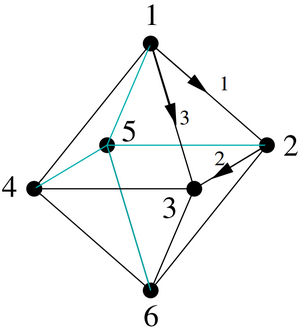# Science:Math Exam Resources/Courses/MATH307/April 2012/Question 04 (d)

MATH307 April 2012

Work in progress: this question page is incomplete, there might be mistakes in the material you are seeing here.
Other MATH307 Exams

### Question 04 (d)

Consider a network arranged in the shape of an octahedron as in this diagram:The nodes have been labeled with the large numbers and 3 of the edges (resistors) have been given orientations and labeled with small numbers. Assume all resistances are equal to 1. Let D be the incidence matrix (for some choice of labeling and arrows for the remaining edges) and let L be the Laplacian.

(d) Write down the Laplacian L.

 Make sure you understand the problem fully: What is the question asking you to do? Are there specific conditions or constraints that you should take note of? How will you know if your answer is correct from your work only? Can you rephrase the question in your own words in a way that makes sense to you? If you are stuck, check the hint below. Consider it for a while. Does it give you a new idea on how to approach the problem? If so, try it!

 Checking a solution serves two purposes: helping you if, after having used the hint, you still are stuck on the problem; or if you have solved the problem and would like to check your work. If you are stuck on a problem: Read the solution slowly and as soon as you feel you could finish the problem on your own, hide it and work on the problem. Come back later to the solution if you are stuck or if you want to check your work. If you want to check your work: Don't only focus on the answer, problems are mostly marked for the work you do, make sure you understand all the steps that were required to complete the problem and see if you made mistakes or forgot some aspects. Your goal is to check that your mental process was correct, not only the result.Math Learning Centre A space to study math together. Free math graduate and undergraduate TA support. Mon - Fri: 11 am - 5 pm, Private tutor We can help to